# RD Sharma Solutions For Class 12 Maths Exercise 2.1 Chapter 2 Function

The PDF of RD Sharma Solutions for Class 12 Maths Chapter 2 Function Exercise 2.1 are provided here. Students who are preparing for their exams and who wish to present their answers without any mistakes can refer to RD Sharma Solutions for Class 12. On regular practice, students can speed up the method of solving problems by using shortcut tips to secure high marks in their examination. Students can download the pdf from the links provided below. This exercise deals with basic concepts related to functions. Some of the important concepts of this exercise are listed below.

• Classification of functions
• Types of functions
• Constant function
• Identity function
• Modulus function
• Integer function
• Exponential function
• Logarithmic function
• Reciprocal function
• Square root function
• Operations on real functions
• Kinds of functions
• One-one function
• Onto function
• Many one function
• In to function
• Bijection

## Download the PDF of RD Sharma Solutions For Class 12 Chapter 2 – Function Exercise 2.1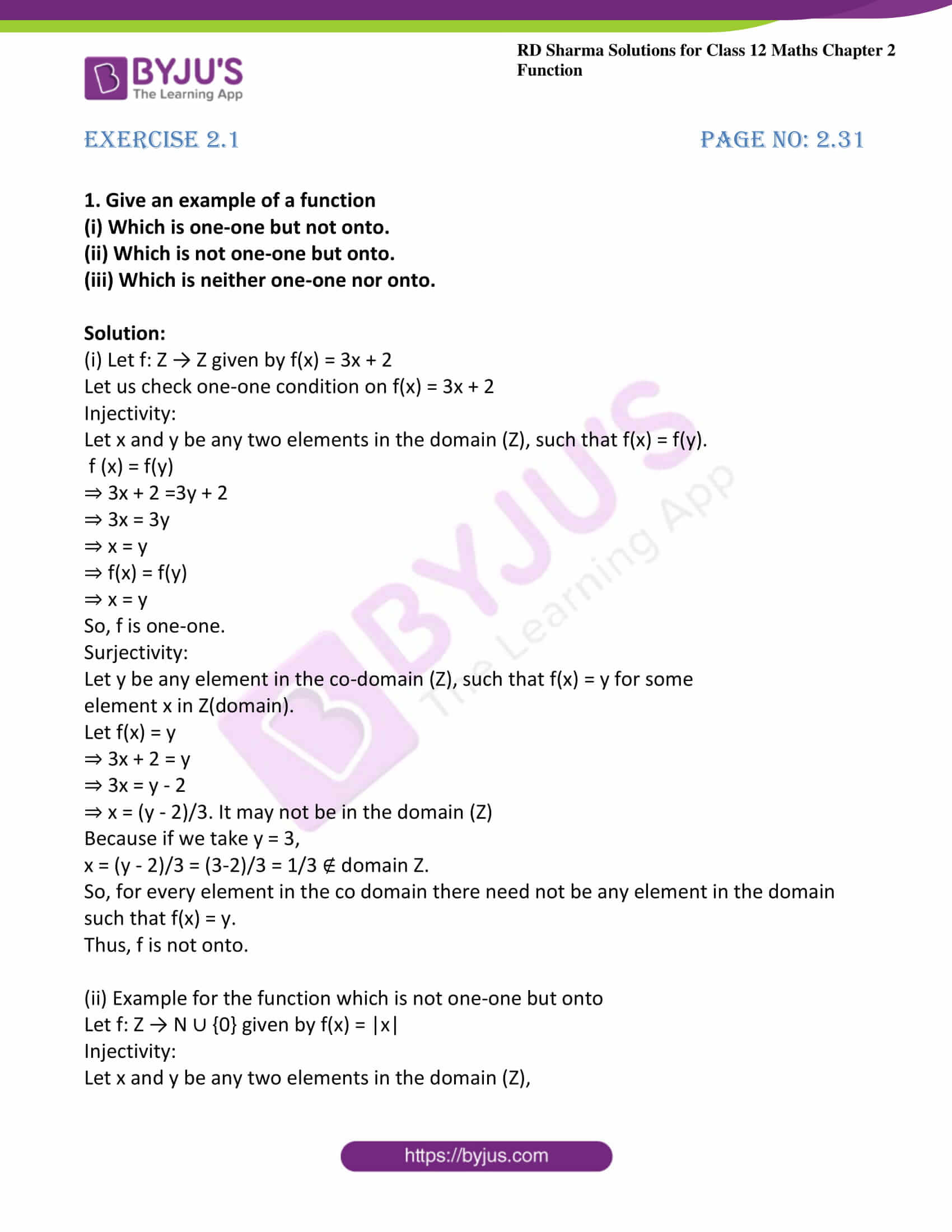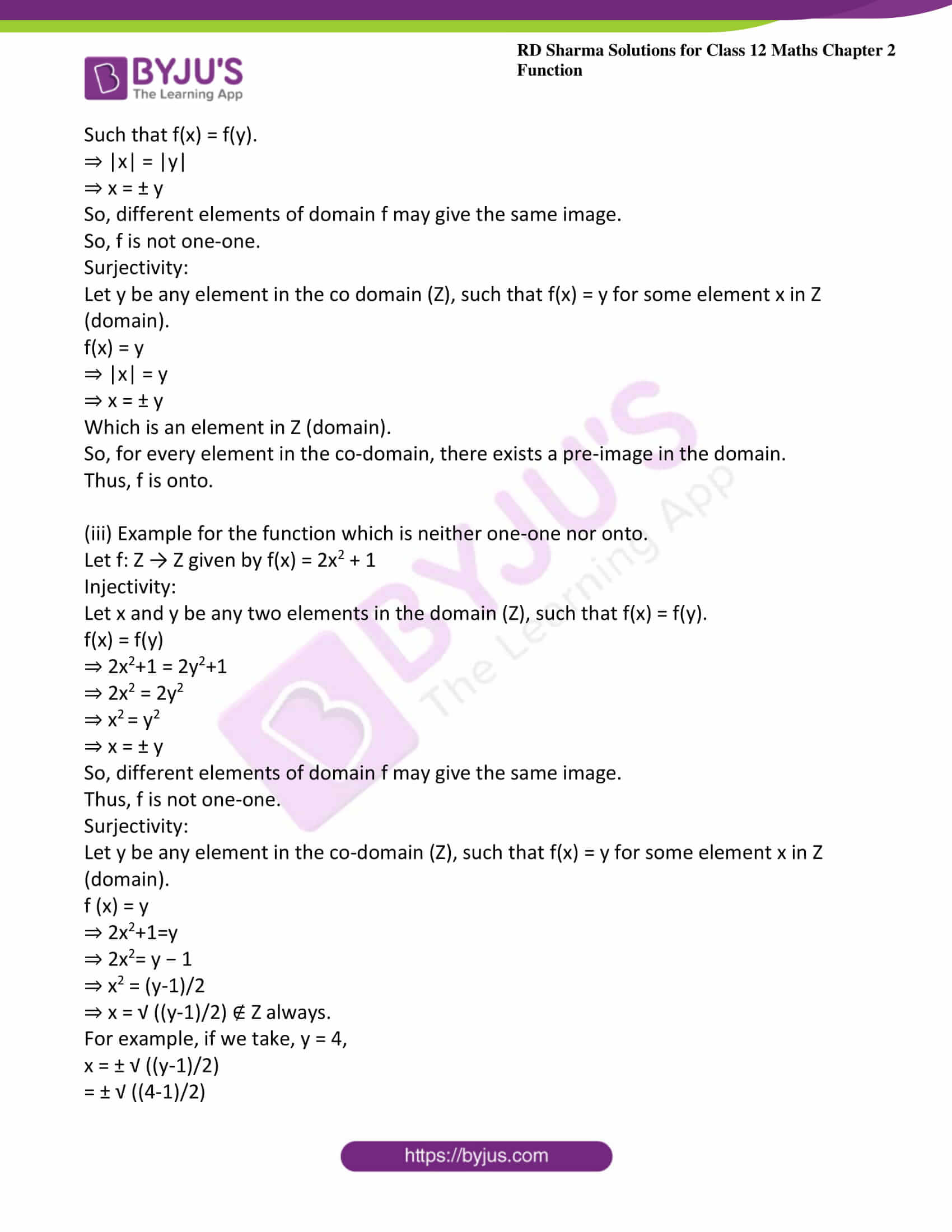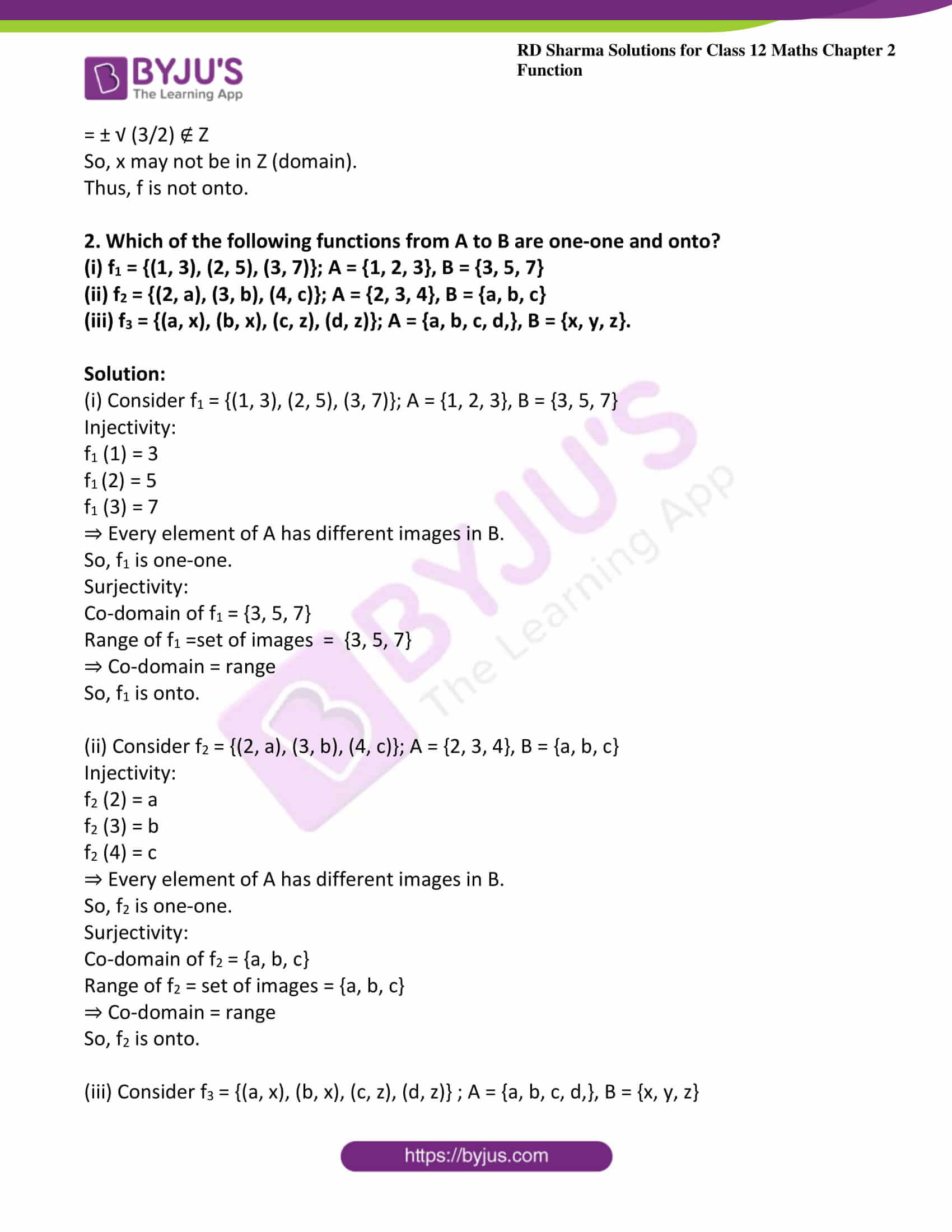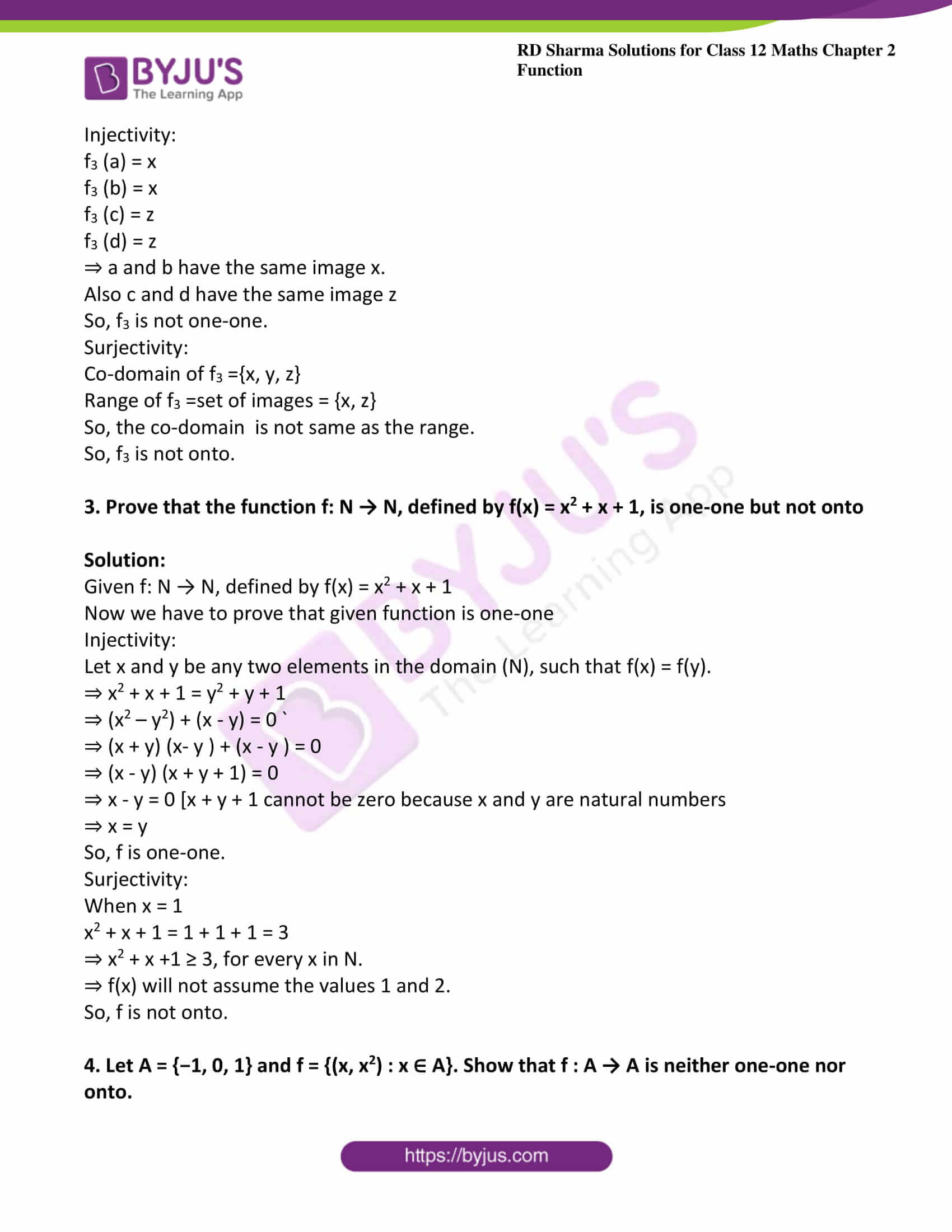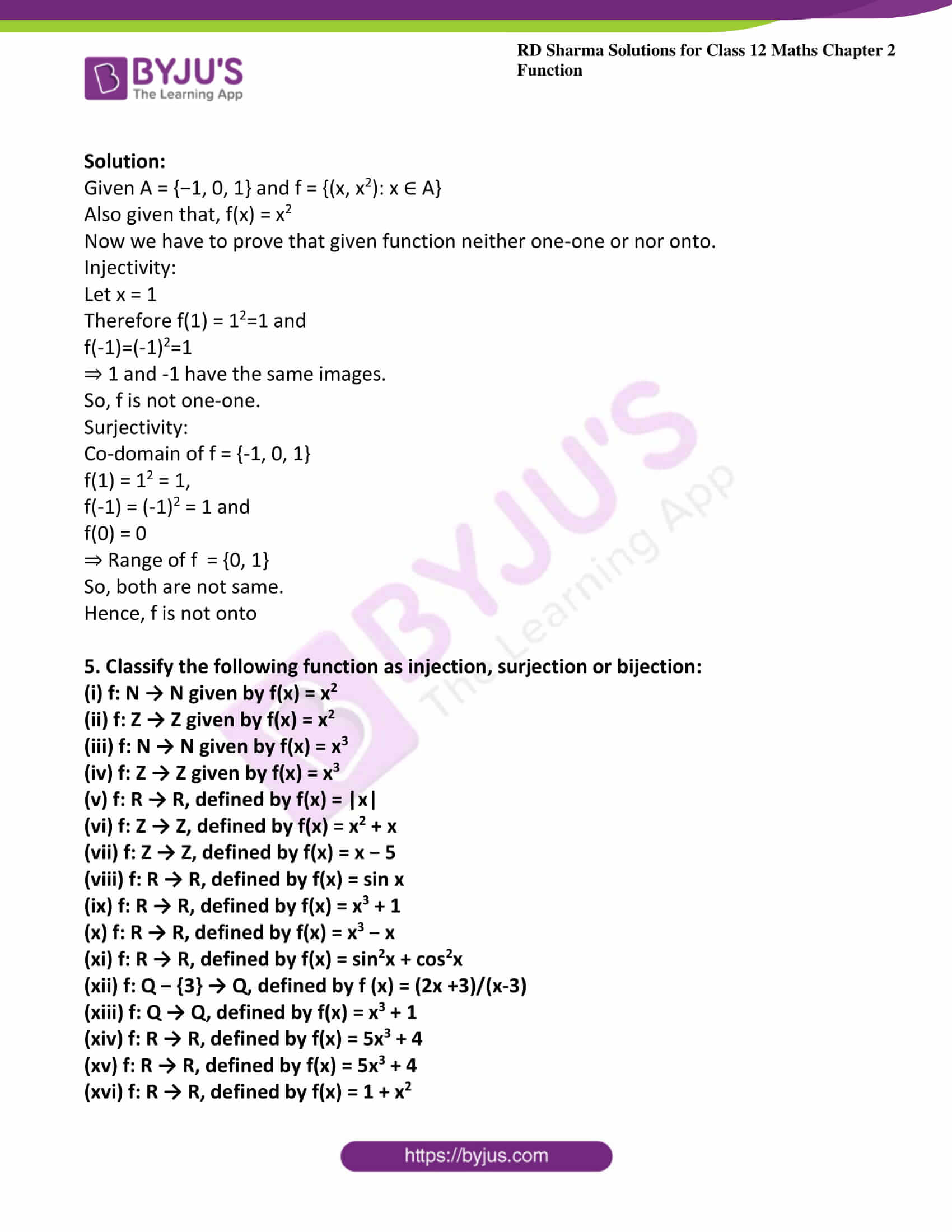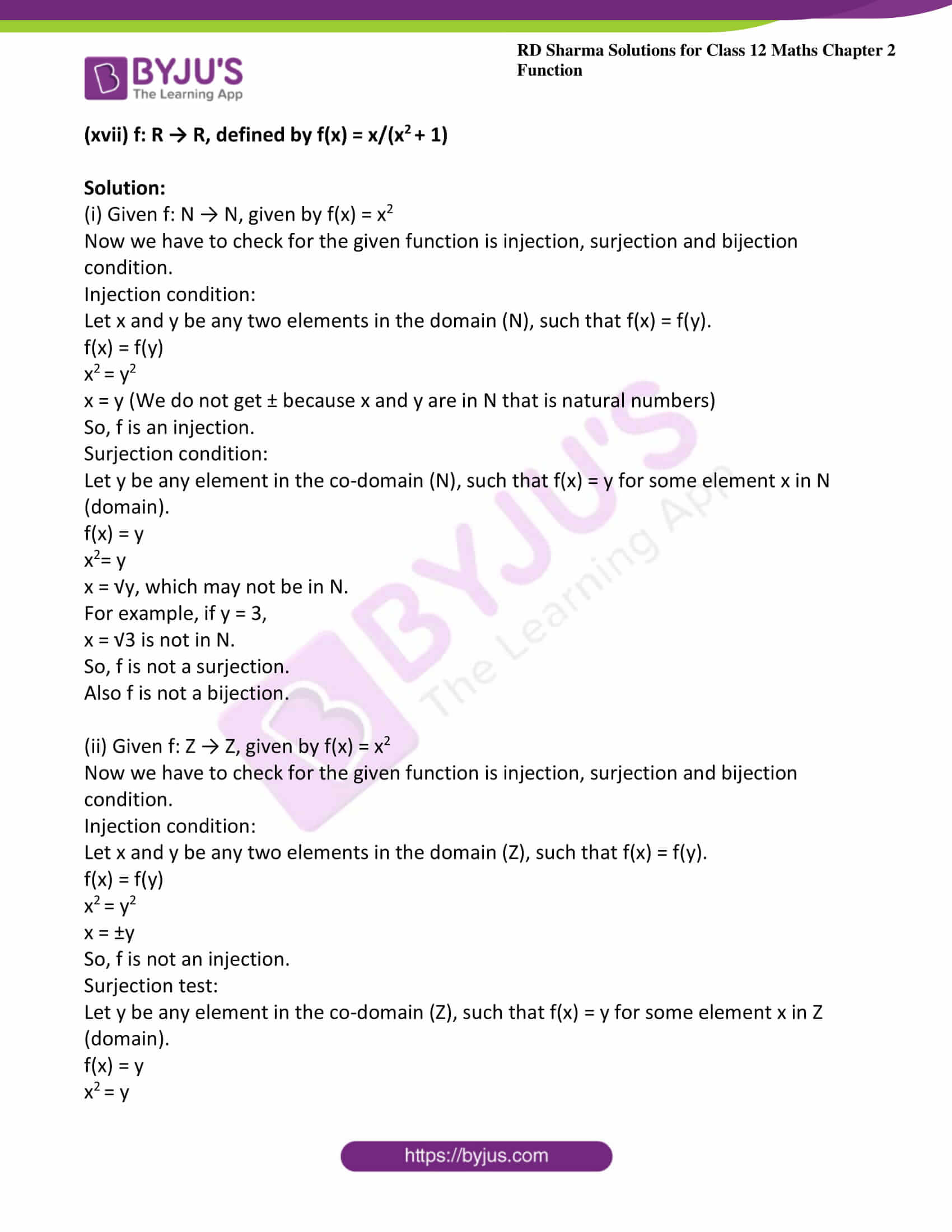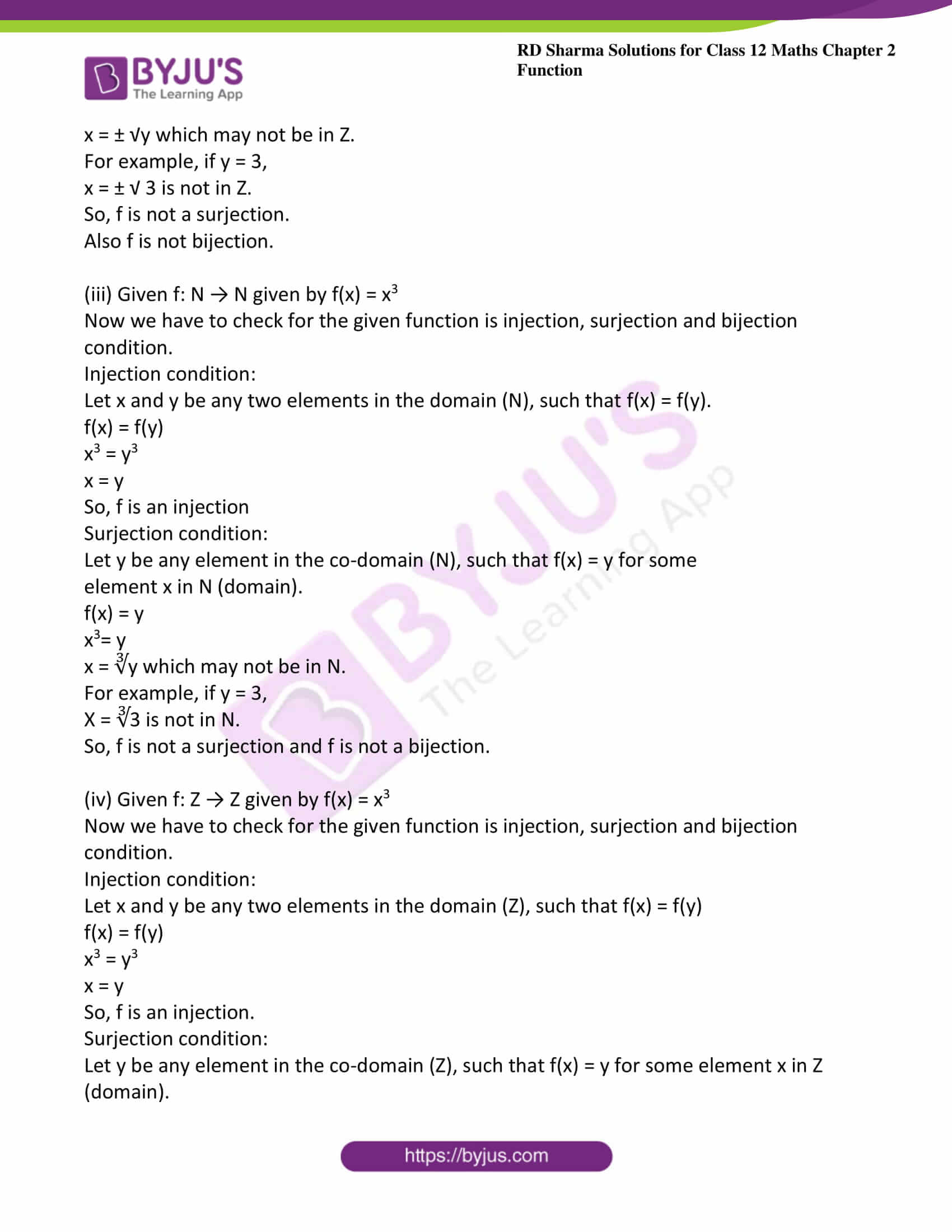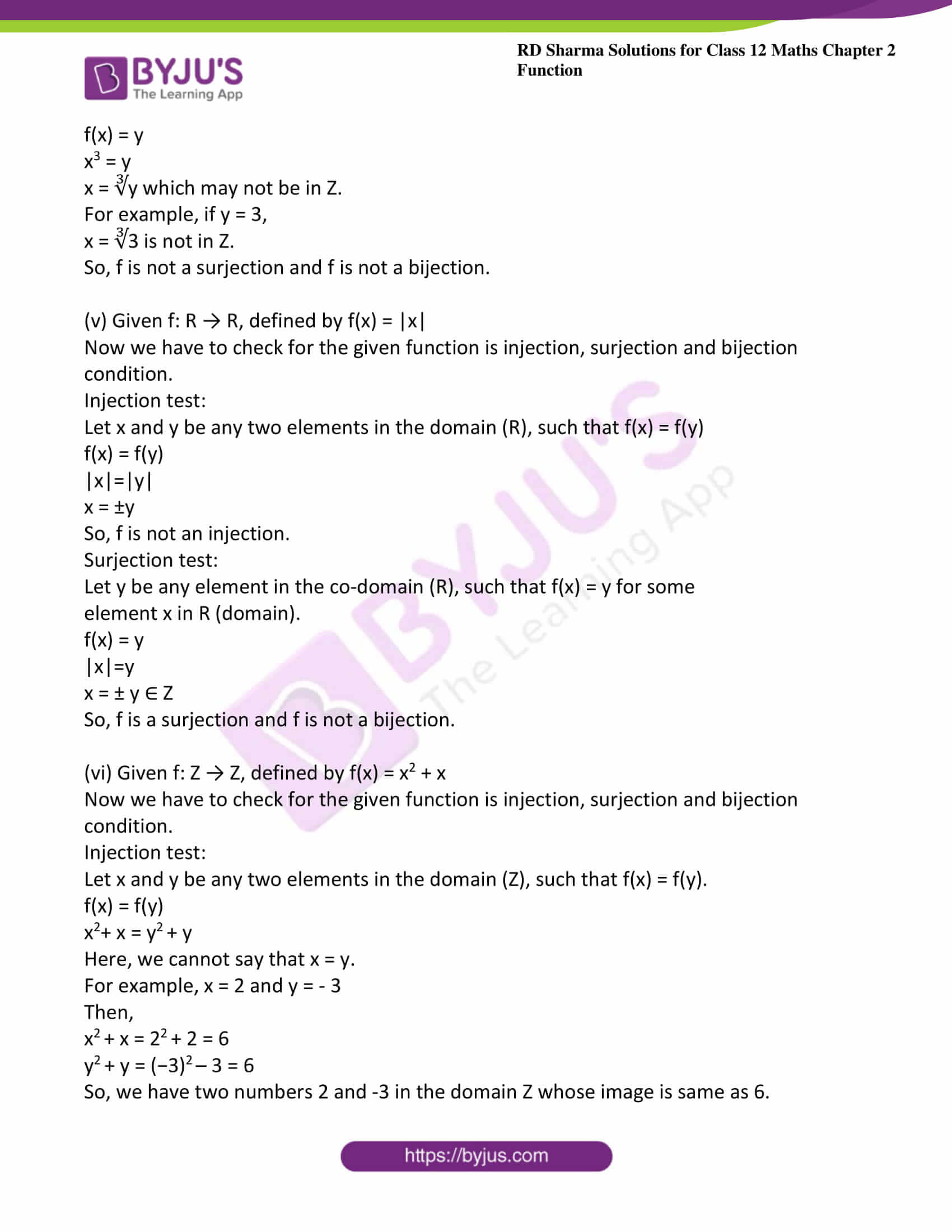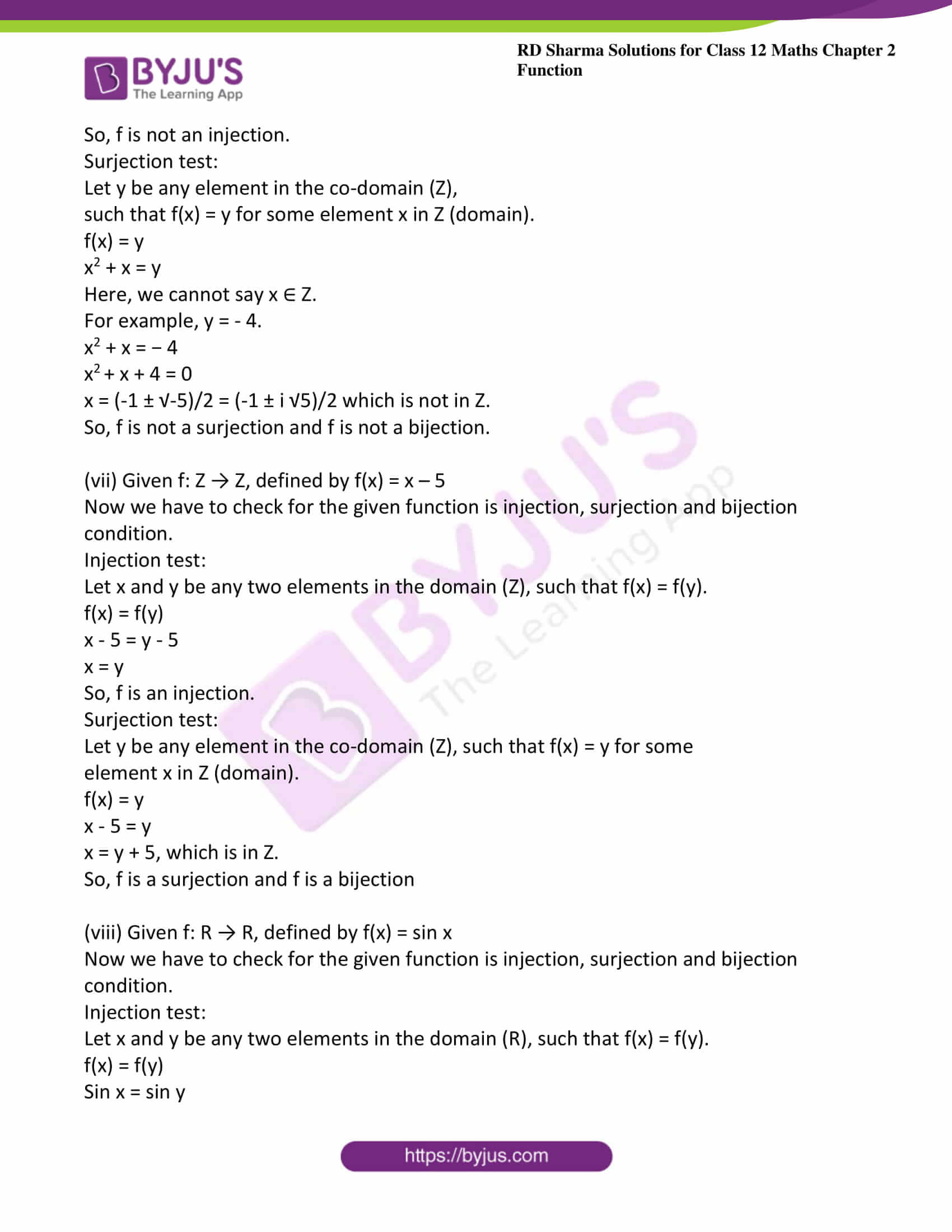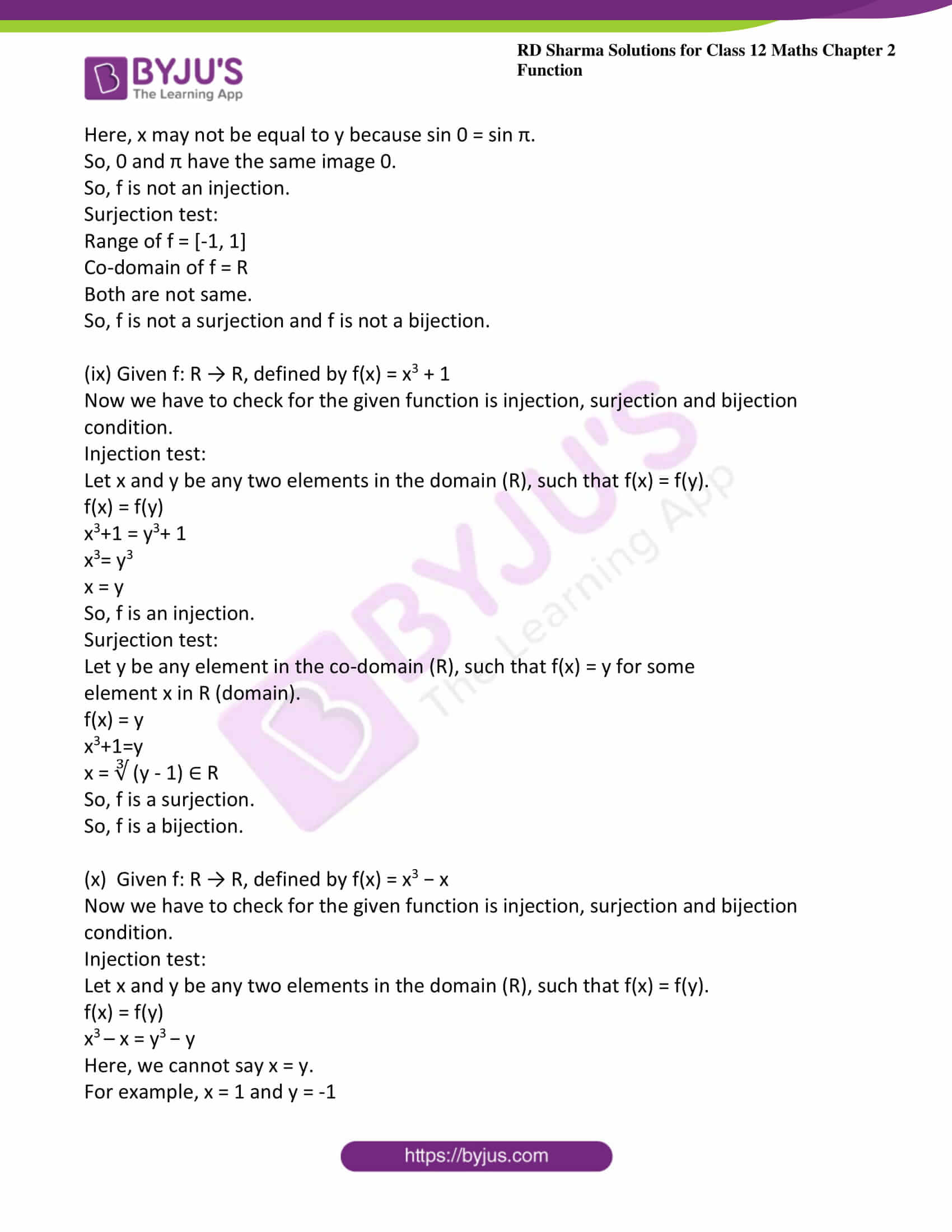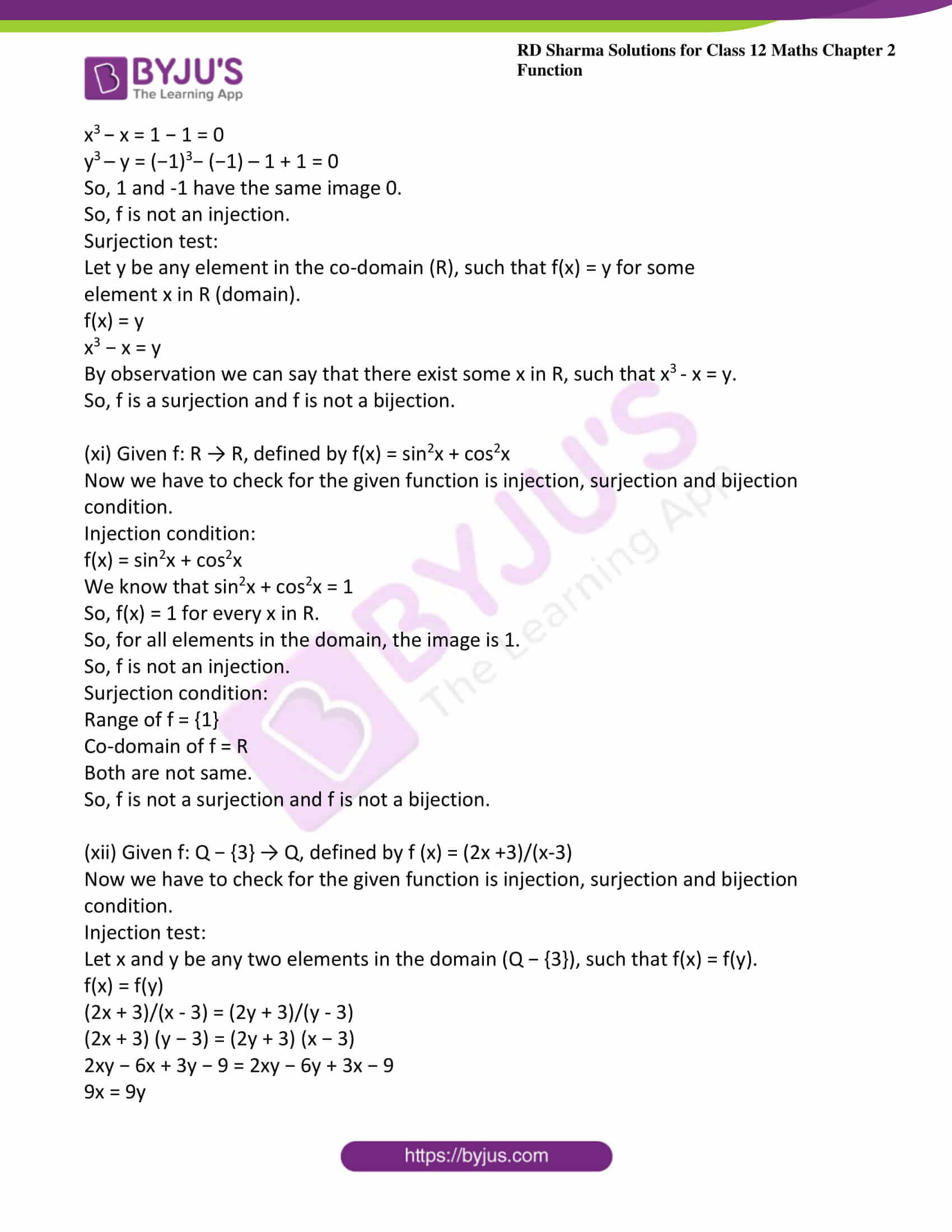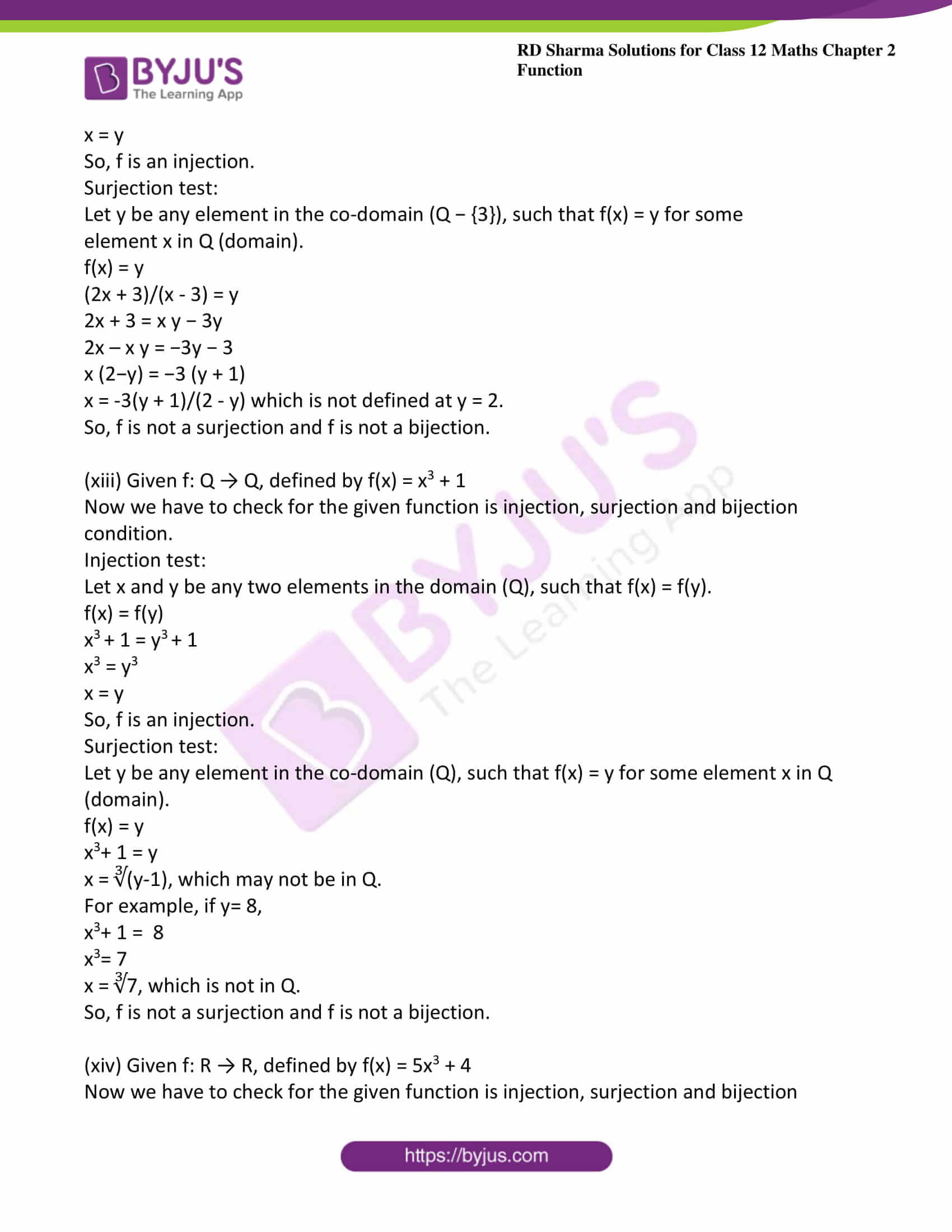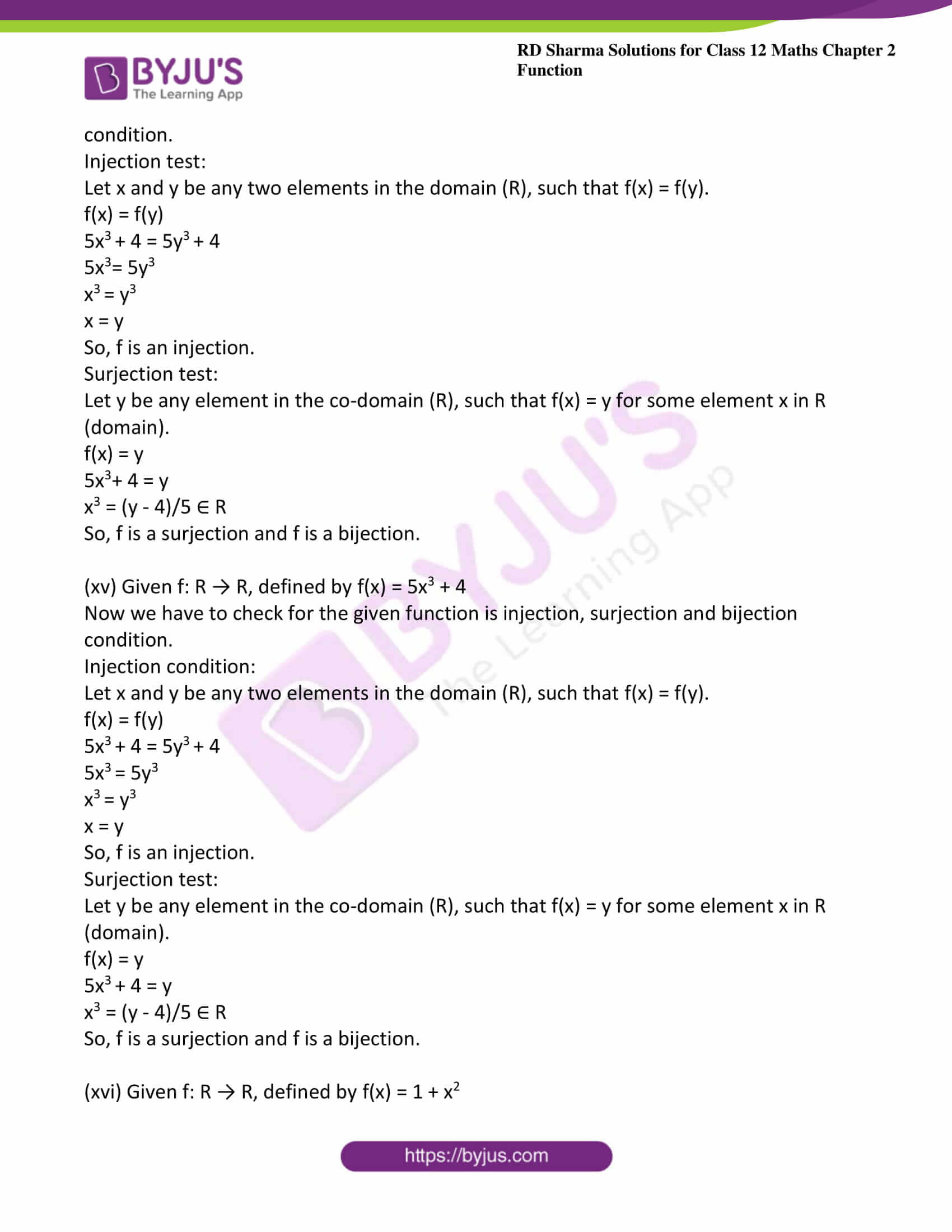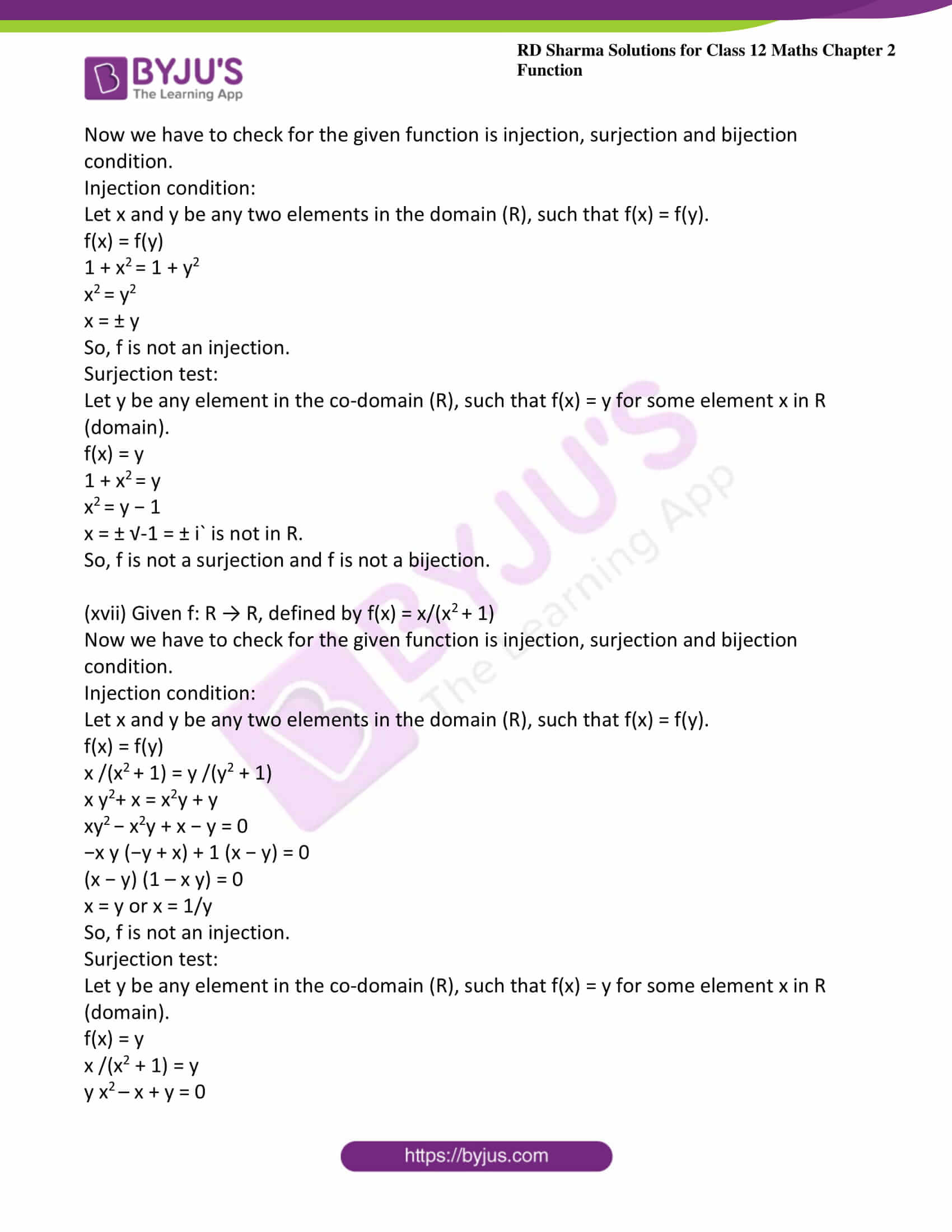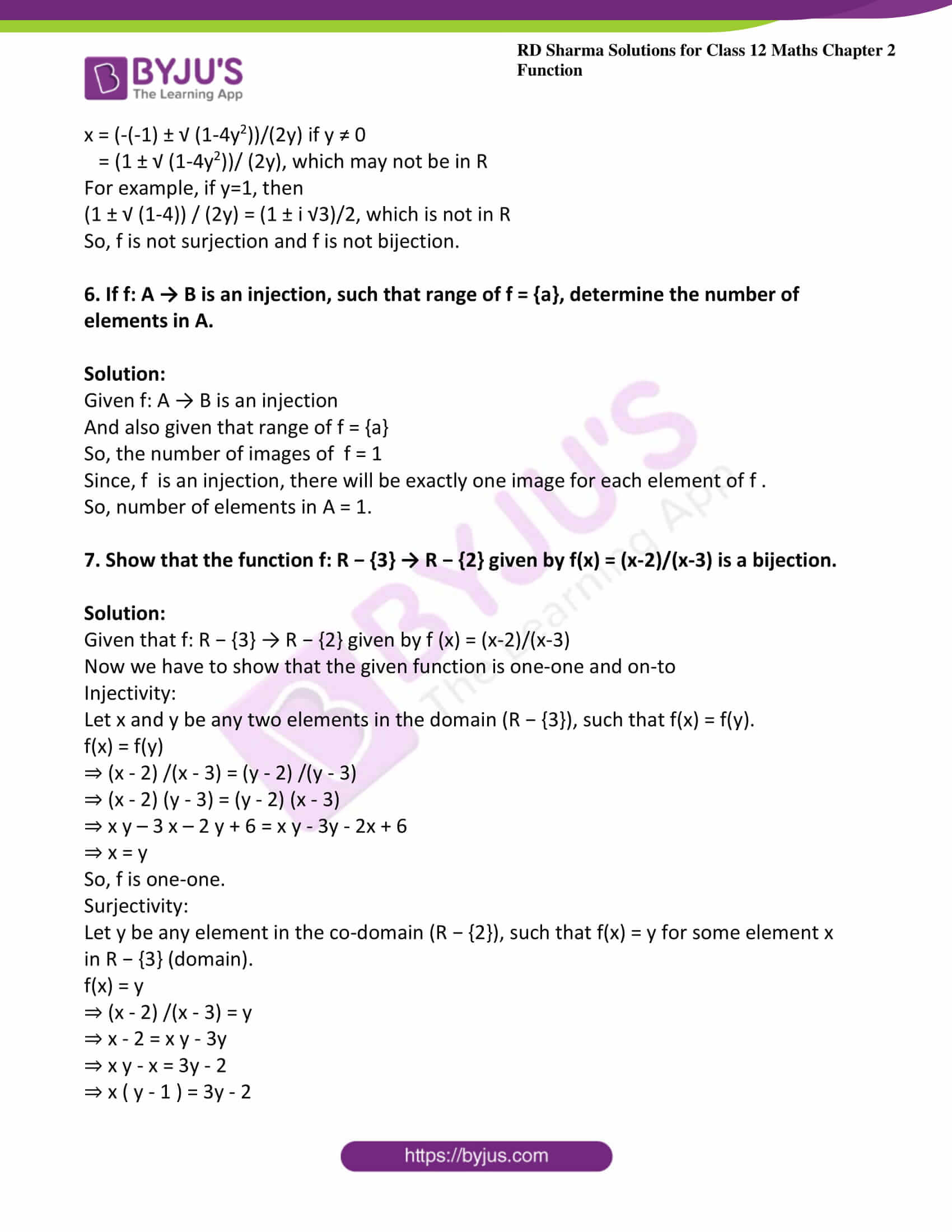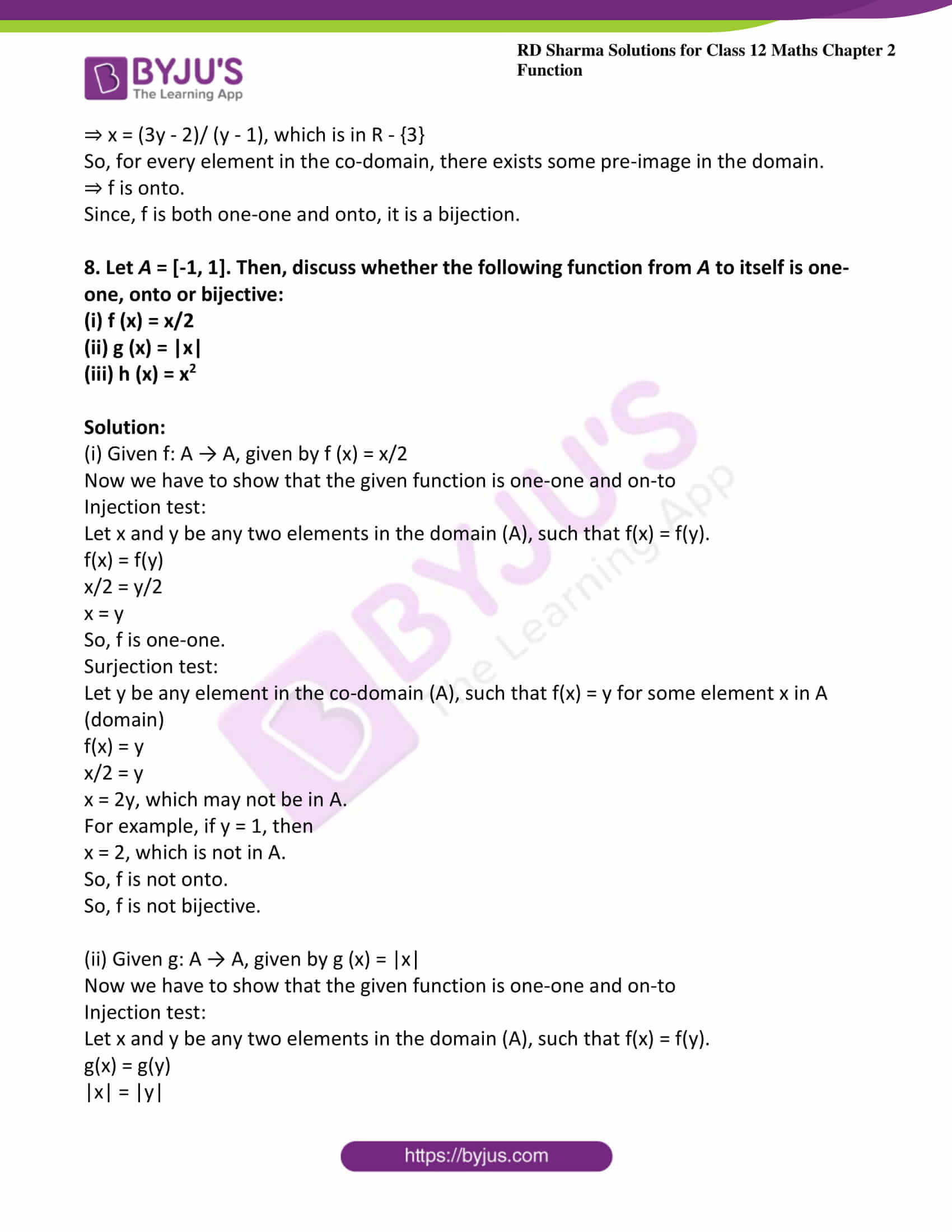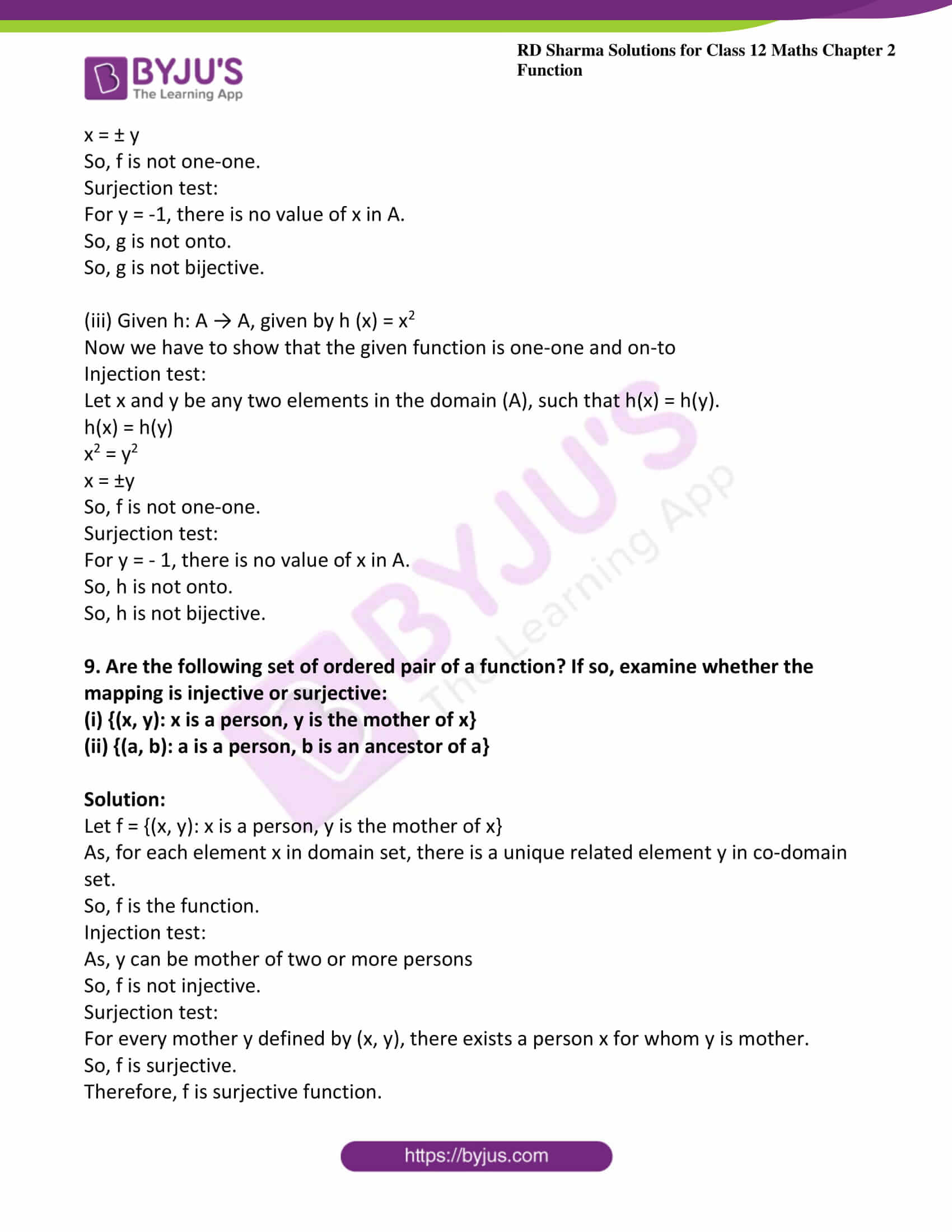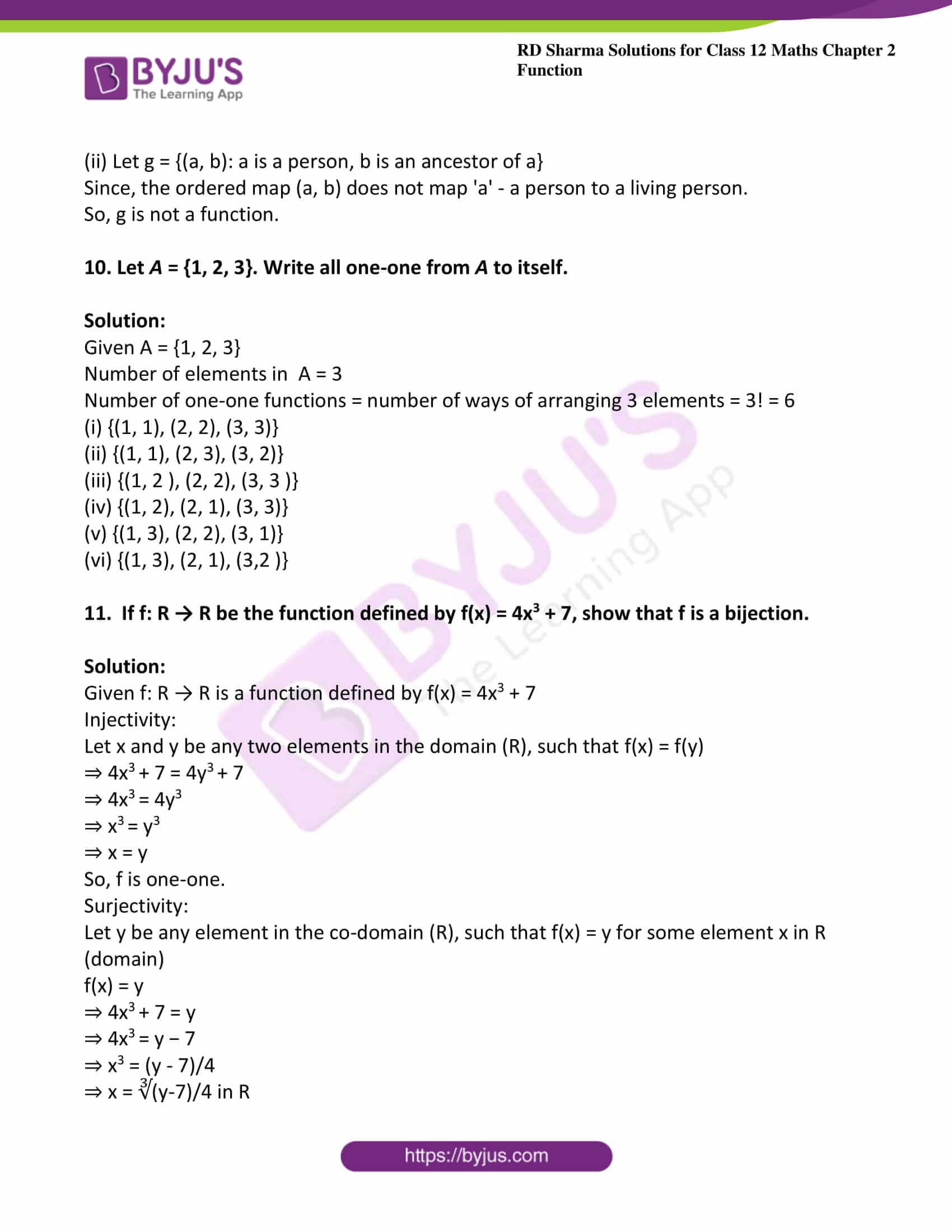### Access other exercises of RD Sharma Solutions For Class 12 Chapter 2 – Function

Exercise 2.2 Solutions

Exercise 2.3 Solutions

Exercise 2.4 Solutions

### Exercise 2.1 Page No: 2.31

1. Give an example of a function

(i) Which is one-one but not onto.

(ii) Which is not one-one but onto.

(iii) Which is neither one-one nor onto.

Solution:

(i) Let f: Z → Z given by f(x) = 3x + 2

Let us check one-one condition on f(x) = 3x + 2

Injectivity:

Let x and y be any two elements in the domain (Z), such that f(x) = f(y).

f (x) = f(y)

⇒ 3x + 2 =3y + 2

⇒ 3x = 3y

⇒ x = y

⇒ f(x) = f(y)

⇒ x = y

So, f is one-one.

Surjectivity:

Let y be any element in the co-domain (Z), such that f(x) = y for some element x in Z(domain).

Let f(x) = y

⇒ 3x + 2 = y

⇒ 3x = y – 2

⇒ x = (y – 2)/3. It may not be in the domain (Z)

Because if we take y = 3,

x = (y – 2)/3 = (3-2)/3 = 1/3 ∉ domain Z.

So, for every element in the co domain there need not be any element in the domain such that f(x) = y.

Thus, f is not onto.

(ii) Example for the function which is not one-one but onto

Let f: Z → N ∪ {0} given by f(x) = |x|

Injectivity:

Let x and y be any two elements in the domain (Z),

Such that f(x) = f(y).

⇒ |x| = |y|

⇒ x = ± y

So, different elements of domain f may give the same image.

So, f is not one-one.

Surjectivity:

Let y be any element in the co domain (Z), such that f(x) = y for some element x in Z (domain).

f(x) = y

⇒ |x| = y

⇒ x = ± y

Which is an element in Z (domain).

So, for every element in the co-domain, there exists a pre-image in the domain.

Thus, f is onto.

(iii) Example for the function which is neither one-one nor onto.

Let f: Z → Z given by f(x) = 2x2 + 1

Injectivity:

Let x and y be any two elements in the domain (Z), such that f(x) = f(y).

f(x) = f(y)

⇒ 2x2+1 = 2y2+1

⇒ 2x2 = 2y2

⇒ x= y2

⇒ x = ± y

So, different elements of domain f may give the same image.

Thus, f is not one-one.

Surjectivity:

Let y be any element in the co-domain (Z), such that f(x) = y for some element x in Z (domain).

f (x) = y

⇒ 2x2+1=y

⇒ 2x2= y − 1

⇒ x2 = (y-1)/2

⇒ x = √ ((y-1)/2) ∉ Z always.

For example, if we take, y = 4,

x = ± √ ((y-1)/2)

= ± √ ((4-1)/2)

= ± √ (3/2) ∉ Z

So, x may not be in Z (domain).

Thus, f is not onto.

2. Which of the following functions from A to B are one-one and onto?
(i) f1 = {(1, 3), (2, 5), (3, 7)}; A = {1, 2, 3}, B = {3, 5, 7}

(ii) f2 = {(2, a), (3, b), (4, c)}; A = {2, 3, 4}, B = {a, b, c}

(iii) f3 = {(a, x), (b, x), (c, z), (d, z)}; A = {a, b, c, d,}, B = {x, y, z}.

Solution:

(i) Consider f1 = {(1, 3), (2, 5), (3, 7)}; A = {1, 2, 3}, B = {3, 5, 7}

Injectivity:

f1 (1) = 3

f(2) = 5

f1 (3) = 7

⇒ Every element of A has different images in B.

So, f1 is one-one.

Surjectivity:

Co-domain of f1 = {3, 5, 7}

Range of f1 =set of images  =  {3, 5, 7}

⇒ Co-domain = range

So, f1 is onto.

(ii) Consider f2 = {(2, a), (3, b), (4, c)}; A = {2, 3, 4}, B = {a, b, c}

Injectivity:

f2 (2) = a

f2 (3) = b

f2 (4) = c

⇒ Every element of A has different images in B.

So, f2 is one-one.

Surjectivity:

Co-domain of f2 = {a, b, c}

Range of f2 = set of images = {a, b, c}

⇒ Co-domain = range

So, f2 is onto.

(iii) Consider f3 = {(a, x), (b, x), (c, z), (d, z)} ; A = {a, b, c, d,}, B = {x, y, z}

Injectivity:

f3 (a) = x

f3 (b) = x

f3 (c) = z

f3 (d) = z

⇒ a and b have the same image x.

Also c and d have the same image z

So, f3 is not one-one.

Surjectivity:

Co-domain of f3 ={x, y, z}

Range of f3 =set of images = {x, z}

So, the co-domain  is not same as the range.

So, f3 is not onto.

3. Prove that the function f: N → N, defined by f(x) = x2 + x + 1, is one-one but not onto

Solution:

Given f: N → N, defined by f(x) = x2 + x + 1

Now we have to prove that given function is one-one

Injectivity:

Let x and y be any two elements in the domain (N), such that f(x) = f(y).

⇒ x2 + x + 1 = y2 + y + 1

⇒ (x2 – y2) + (x – y) = 0 

⇒ (x + y) (x- y ) + (x – y ) = 0

⇒ (x – y) (x + y + 1) = 0

⇒ x – y = 0 [x + y + 1 cannot be zero because x and y are natural numbers

⇒ x = y

So, f is one-one.

Surjectivity:

When x = 1

x2 + x + 1 = 1 + 1 + 1 = 3

⇒ x2 + x +1 ≥ 3, for every x in N.

⇒ f(x) will not assume the values 1 and 2.

So, f is not onto.

4. Let A = {−1, 0, 1} and f = {(x, x2) : x ∈ A}. Show that f : A → A is neither one-one nor onto.

Solution:

Given A = {−1, 0, 1} and f = {(x, x2): x ∈ A}

Also given that, f(x) = x2

Now we have to prove that given function neither one-one or nor onto.

Injectivity:

Let x = 1

Therefore f(1) = 12=1 and

f(-1)=(-1)2=1

⇒ 1 and -1 have the same images.

So, f is not one-one.

Surjectivity:

Co-domain of f = {-1, 0, 1}

f(1) = 12 = 1,

f(-1) = (-1)2 = 1 and

f(0) = 0

⇒ Range of f  = {0, 1}

So, both are not same.

Hence, f is not onto

5. Classify the following function as injection, surjection or bijection:

(i) f: N → N given by f(x) = x2

(ii) f: Z → Z given by f(x) = x2

(iii) f: N → N given by f(x) = x3

(iv) f: Z → Z given by f(x) = x3

(v) f: R → R, defined by f(x) = |x|

(vi) f: Z → Z, defined by f(x) = x2 + x

(vii) f: Z → Z, defined by f(x) = x − 5

(viii) f: R → R, defined by f(x) = sin x

(ix) f: R → R, defined by f(x) = x3 + 1

(x) f: R → R, defined by f(x) = x3 − x

(xi) f: R → R, defined by f(x) = sin2x + cos2x

(xii) f: Q − {3} → Q, defined by f (x) = (2x +3)/(x-3)

(xiii) f: Q → Q, defined by f(x) = x3 + 1

(xiv) f: R → R, defined by f(x) = 5x3 + 4

(xv) f: R → R, defined by f(x) = 5x3 + 4

(xvi) f: R → R, defined by f(x) = 1 + x2

(xvii) f: R → R, defined by f(x) = x/(x2 + 1)

Solution:

(i) Given f: N → N, given by f(x) = x2

Now we have to check for the given function is injection, surjection and bijection condition.

Injection condition:

Let x and y be any two elements in the domain (N), such that f(x) = f(y).

f(x) = f(y)

x2 = y2

x = y (We do not get ± because x and y are in N that is natural numbers)

So, f is an injection.

Surjection condition:

Let y be any element in the co-domain (N), such that f(x) = y for some element x in N (domain).

f(x) = y

x2= y

x = √y, which may not be in N.

For example, if y = 3,

x = √3 is not in N.

So, f is not a surjection.

Also f is not a bijection.

(ii) Given f: Z → Z, given by f(x) = x2

Now we have to check for the given function is injection, surjection and bijection condition.

Injection condition:

Let x and y be any two elements in the domain (Z), such that f(x) = f(y).

f(x) = f(y)

x2 = y2

x = ±y

So, f is not an injection.

Surjection test:

Let y be any element in the co-domain (Z), such that f(x) = y for some element x in Z (domain).

f(x) = y

x2 = y

x = ± √y which may not be in Z.

For example, if y = 3,

x = ± √ 3 is not in Z.

So, f is not a surjection.

Also f is not bijection.

(iii) Given f: N → N given by f(x) = x3

Now we have to check for the given function is injection, surjection and bijection condition.

Injection condition:

Let x and y be any two elements in the domain (N), such that f(x) = f(y).

f(x) = f(y)

x3 = y3

x = y

So, f is an injection

Surjection condition:

Let y be any element in the co-domain (N), such that f(x) = y for some element x in N (domain).

f(x) = y

x3= y

x = ∛y which may not be in N.

For example, if y = 3,

X = ∛3 is not in N.

So, f is not a surjection and f is not a bijection.

(iv) Given f: Z → Z given by f(x) = x3

Now we have to check for the given function is injection, surjection and bijection condition.

Injection condition:

Let x and y be any two elements in the domain (Z), such that f(x) = f(y)

f(x) = f(y)

x3 = y3

x = y

So, f is an injection.

Surjection condition:

Let y be any element in the co-domain (Z), such that f(x) = y for some element x in Z (domain).

f(x) = y

x3 = y

x = ∛y which may not be in Z.

For example, if y = 3,

x = ∛3 is not in Z.

So, f is not a surjection and f is not a bijection.

(v) Given f: R → R, defined by f(x) = |x|

Now we have to check for the given function is injection, surjection and bijection condition.

Injection test:

Let x and y be any two elements in the domain (R), such that f(x) = f(y)

f(x) = f(y)

|x|=|y|

x = ±y

So, f is not an injection.

Surjection test:

Let y be any element in the co-domain (R), such that f(x) = y for some element x in R (domain).

f(x) = y

|x|=y

x = ± y ∈ Z

So, f is a surjection and f is not a bijection.

(vi) Given f: Z → Z, defined by f(x) = x2 + x

Now we have to check for the given function is injection, surjection and bijection condition.

Injection test:

Let x and y be any two elements in the domain (Z), such that f(x) = f(y).

f(x) = f(y)

x2+ x = y+ y

Here, we cannot say that x = y.

For example, x = 2 and y = – 3

Then,

x2 + x = 22 + 2 = 6

y2 + y = (−3)2 – 3 = 6

So, we have two numbers 2 and -3 in the domain Z whose image is same as 6.

So, f is not an injection.

Surjection test:

Let y be any element in the co-domain (Z),

such that f(x) = y for some element x in Z (domain).

f(x) = y

x2 + x = y

Here, we cannot say x ∈ Z.

For example, y = – 4.

x2 + x = − 4

x2 + x + 4 = 0

x = (-1 ± √-5)/2 = (-1 ± i √5)/2 which is not in Z.

So, f is not a surjection and f is not a bijection.

(vii) Given f: Z → Z, defined by f(x) = x – 5

Now we have to check for the given function is injection, surjection and bijection condition.

Injection test:

Let x and y be any two elements in the domain (Z), such that f(x) = f(y).

f(x) = f(y)

x – 5 = y – 5

x = y

So, f is an injection.

Surjection test:

Let y be any element in the co-domain (Z), such that f(x) = y for some element x in Z (domain).

f(x) = y

x – 5 = y

x = y + 5, which is in Z.

So, f is a surjection and f is a bijection

(viii) Given f: R → R, defined by f(x) = sin x

Now we have to check for the given function is injection, surjection and bijection condition.

Injection test:

Let x and y be any two elements in the domain (R), such that f(x) = f(y).

f(x) = f(y)

Sin x = sin y

Here, x may not be equal to y because sin 0 = sin π.

So, 0 and π have the same image 0.

So, f is not an injection.

Surjection test:

Range of f = [-1, 1]

Co-domain of f = R

Both are not same.

So, f is not a surjection and f is not a bijection.

(ix) Given f: R → R, defined by f(x) = x3 + 1

Now we have to check for the given function is injection, surjection and bijection condition.

Injection test:

Let x and y be any two elements in the domain (R), such that f(x) = f(y).

f(x) = f(y)

x3+1 = y3+ 1

x3= y3

x = y

So, f is an injection.

Surjection test:

Let y be any element in the co-domain (R), such that f(x) = y for some element x in R (domain).

f(x) = y

x3+1=y

x = ∛ (y – 1) ∈ R

So, f is a surjection.

So, f is a bijection.

(x)  Given f: R → R, defined by f(x) = x3 − x

Now we have to check for the given function is injection, surjection and bijection condition.

Injection test:

Let x and y be any two elements in the domain (R), such that f(x) = f(y).

f(x) = f(y)

x3 – x = y3 − y

Here, we cannot say x = y.

For example, x = 1 and y = -1

x3 − x = 1 − 1 = 0

y3 – y = (−1)3− (−1) – 1 + 1 = 0

So, 1 and -1 have the same image 0.

So, f is not an injection.

Surjection test:

Let y be any element in the co-domain (R), such that f(x) = y for some element x in R (domain).

f(x) = y

x3 − x = y

By observation we can say that there exist some x in R, such that x– x = y.

So, f is a surjection and f is not a bijection.

(xi) Given f: R → R, defined by f(x) = sin2x + cos2x

Now we have to check for the given function is injection, surjection and bijection condition.

Injection condition:

f(x) = sin2x + cos2x

We know that sin2x + cos2x = 1

So, f(x) = 1 for every x in R.

So, for all elements in the domain, the image is 1.

So, f is not an injection.

Surjection condition:

Range of f = {1}

Co-domain of f = R

Both are not same.

So, f is not a surjection and f is not a bijection.

(xii) Given f: Q − {3} → Q, defined by f (x) = (2x +3)/(x-3)

Now we have to check for the given function is injection, surjection and bijection condition.

Injection test:

Let x and y be any two elements in the domain (Q − {3}), such that f(x) = f(y).

f(x) = f(y)

(2x + 3)/(x – 3) = (2y + 3)/(y – 3)

(2x + 3) (y − 3) = (2y + 3) (x − 3)

2xy − 6x + 3y − 9 = 2xy − 6y + 3x − 9

9x = 9y

x = y

So, f is an injection.

Surjection test:

Let y be any element in the co-domain (Q − {3}), such that f(x) = y for some element x in Q (domain).

f(x) = y

(2x + 3)/(x – 3) = y

2x + 3 = x y − 3y

2x – x y = −3y − 3

x (2−y) = −3 (y + 1)

x = -3(y + 1)/(2 – y) which is not defined at y = 2.

So, f is not a surjection and f is not a bijection.

(xiii) Given f: Q → Q, defined by f(x) = x3 + 1

Now we have to check for the given function is injection, surjection and bijection condition.

Injection test:

Let x and y be any two elements in the domain (Q), such that f(x) = f(y).

f(x) = f(y)

x3 + 1 = y3 + 1

x3 = y3

x = y

So, f is an injection.

Surjection test:

Let y be any element in the co-domain (Q), such that f(x) = y for some element x in Q (domain).

f(x) = y

x3+ 1 = y

x = ∛(y-1), which may not be in Q.

For example, if y= 8,

x3+ 1 =  8

x3= 7

x = ∛7, which is not in Q.

So, f is not a surjection and f is not a bijection.

(xiv) Given f: R → R, defined by f(x) = 5x3 + 4

Now we have to check for the given function is injection, surjection and bijection condition.

Injection test:

Let x and y be any two elements in the domain (R), such that f(x) = f(y).

f(x) = f(y)

5x3 + 4 = 5y3 + 4

5x3= 5y3

x3 = y3

x = y

So, f is an injection.

Surjection test:

Let y be any element in the co-domain (R), such that f(x) = y for some element x in R (domain).

f(x) = y

5x3+ 4 = y

x3 = (y – 4)/5 ∈ R

So, f is a surjection and f is a bijection.

(xv) Given f: R → R, defined by f(x) = 5x3 + 4

Now we have to check for the given function is injection, surjection and bijection condition.

Injection condition:

Let x and y be any two elements in the domain (R), such that f(x) = f(y).

f(x) = f(y)

5x3 + 4 = 5y3 + 4

5x3 = 5y3

x3 = y3

x = y

So, f is an injection.

Surjection test:

Let y be any element in the co-domain (R), such that f(x) = y for some element x in R (domain).

f(x) = y

5x3 + 4 = y

x3 = (y – 4)/5 ∈ R

So, f is a surjection and f is a bijection.

(xvi) Given f: R → R, defined by f(x) = 1 + x2

Now we have to check for the given function is injection, surjection and bijection condition.

Injection condition:

Let x and y be any two elements in the domain (R), such that f(x) = f(y).

f(x) = f(y)

1 + x2 = 1 + y2

x= y2

x = ± y

So, f is not an injection.

Surjection test:

Let y be any element in the co-domain (R), such that f(x) = y for some element x in R (domain).

f(x) = y

1 + x2 = y

x2 = y − 1

x = ± √-1 = ± i is not in R.

So, f is not a surjection and f is not a bijection.

(xvii) Given f: R → R, defined by f(x) = x/(x2 + 1)

Now we have to check for the given function is injection, surjection and bijection condition.

Injection condition:

Let x and y be any two elements in the domain (R), such that f(x) = f(y).

f(x) = f(y)

x /(x2 + 1) = y /(y2 + 1)

x y2+ x = x2y + y

xy2 − x2y + x − y = 0

−x y (−y + x) + 1 (x − y) = 0

(x − y) (1 – x y) = 0

x = y or x = 1/y

So, f is not an injection.

Surjection test:

Let y be any element in the co-domain (R), such that f(x) = y for some element x in R (domain).

f(x) = y

x /(x2 + 1) = y

y x2 – x + y = 0

x = (-(-1) ± √ (1-4y2))/(2y) if y ≠ 0

= (1 ± √ (1-4y2))/ (2y), which may not be in R

For example, if y=1, then

(1 ± √ (1-4)) / (2y) = (1 ± i √3)/2, which is not in R

So, f is not surjection and f is not bijection.

6. If f: A → B is an injection, such that range of f = {a}, determine the number of elements in A.

Solution:

Given f: A → B is an injection

And also given that range of f = {a}

So, the number of images of  f = 1

Since, f  is an injection, there will be exactly one image for each element of f .

So, number of elements in A = 1.

7. Show that the function f: R − {3} → R − {2} given by f(x) = (x-2)/(x-3) is a bijection.

Solution:

Given that f: R − {3} → R − {2} given by f (x) = (x-2)/(x-3)

Now we have to show that the given function is one-one and on-to

Injectivity:

Let x and y be any two elements in the domain (R − {3}), such that f(x) = f(y).

f(x) = f(y)

⇒ (x – 2) /(x – 3) = (y – 2) /(y – 3)

⇒ (x – 2) (y – 3) = (y – 2) (x – 3)

⇒ x y – 3 x – 2 y + 6 = x y – 3y – 2x + 6

⇒ x = y

So, f is one-one.

Surjectivity:

Let y be any element in the co-domain (R − {2}), such that f(x) = y for some element x in R − {3} (domain).

f(x) = y

⇒ (x – 2) /(x – 3) = y

⇒ x – 2 = x y – 3y

⇒ x y – x = 3y – 2

⇒ x ( y – 1 ) = 3y – 2

⇒ x = (3y – 2)/ (y – 1), which is in R – {3}

So, for every element in the co-domain, there exists some pre-image in the domain.

⇒ f is onto.

Since, f is both one-one and onto, it is a bijection.

8. Let A = [-1, 1]. Then, discuss whether the following function from A to itself is one-one, onto or bijective:

(i) f (x) = x/2

(ii) g (x) = |x|

(iii) h (x) = x2

Solution:

(i) Given f: A → A, given by f (x) = x/2

Now we have to show that the given function is one-one and on-to

Injection test:

Let x and y be any two elements in the domain (A), such that f(x) = f(y).

f(x) = f(y)

x/2 = y/2

x = y

So, f is one-one.

Surjection test:

Let y be any element in the co-domain (A), such that f(x) = y for some element x in A (domain)

f(x) = y

x/2 = y

x = 2y, which may not be in A.

For example, if y = 1, then

x = 2, which is not in A.

So, f is not onto.

So, f is not bijective.

(ii) Given g: A → A, given by g (x) = |x|

Now we have to show that the given function is one-one and on-to

Injection test:

Let x and y be any two elements in the domain (A), such that f(x) = f(y).

g(x) = g(y)

|x| = |y|

x = ± y

So, f is not one-one.

Surjection test:

For y = -1, there is no value of x in A.

So, g is not onto.

So, g is not bijective.

(iii) Given h: A → A, given by h (x) = x2

Now we have to show that the given function is one-one and on-to

Injection test:

Let x and y be any two elements in the domain (A), such that h(x) = h(y).

h(x) = h(y)

x2 = y2

x = ±y

So, f is not one-one.

Surjection test:

For y = – 1, there is no value of x in A.

So, h is not onto.

So, h is not bijective.

9. Are the following set of ordered pair of a function? If so, examine whether the mapping is injective or surjective:

(i) {(x, y): x is a person, y is the mother of x}

(ii) {(a, b): a is a person, b is an ancestor of a}

Solution:

Let f = {(x, y): x is a person, y is the mother of x}

As, for each element x in domain set, there is a unique related element y in co-domain set.

So, f is the function.

Injection test:

As, y can be mother of two or more persons

So, f is not injective.

Surjection test:

For every mother y defined by (x, y), there exists a person x for whom y is mother.

So, f is surjective.

Therefore, f is surjective function.

(ii) Let g = {(a, b): a is a person, b is an ancestor of a}

Since, the ordered map (a, b) does not map ‘a’ – a person to a living person.

So, g is not a function.

10. Let A = {1, 2, 3}. Write all one-one from A to itself.

Solution:

Given A = {1, 2, 3}

Number of elements in  A = 3

Number of one-one functions = number of ways of arranging 3 elements = 3! = 6

(i) {(1, 1), (2, 2), (3, 3)}

(ii) {(1, 1), (2, 3), (3, 2)}

(iii) {(1, 2 ), (2, 2), (3, 3 )}

(iv) {(1, 2), (2, 1), (3, 3)}

(v) {(1, 3), (2, 2), (3, 1)}

(vi) {(1, 3), (2, 1), (3,2 )}

11. If f: R → R be the function defined by f(x) = 4x3 + 7, show that f is a bijection.

Solution:

Given f: R → R is a function defined by f(x) = 4x3 + 7

Injectivity:

Let x and y be any two elements in the domain (R), such that f(x) = f(y)

⇒ 4x3 + 7 = 4y3 + 7

⇒ 4x3 = 4y3

⇒ x3 = y3

⇒ x = y

So, f is one-one.

Surjectivity:

Let y be any element in the co-domain (R), such that f(x) = y for some element x in R (domain)

f(x) = y

⇒ 4x3 + 7 = y

⇒ 4x3 = y − 7

⇒ x3 = (y – 7)/4

⇒ x = ∛(y-7)/4 in R

So, for every element in the co-domain, there exists some pre-image in the domain. f is onto.

Since, f is both one-to-one and onto, it is a bijection.